Home | | Electronic Circuits I | Fixed Bias (Base Resistor Bias)

# Fixed Bias (Base Resistor Bias)

The Figure shows the fixed bias circuit. It is the simplest d.c. bias configuration.

Fixed Bias (Base Resistor Bias)The Figure shows the fixed bias circuit. It is the simplest d.c. bias configuration. For the d.c. analysis we can replace capacitor with an open circuit because the reactance of a capacitor for d.c. isIn the base circuit,

Apply KVL, we get

VCC = IBRB + VBE

Therefore,

IB = (VCC - VBE)/RB

For a given transistor, VBE does not vary significantly during use. As VCC is of fixed value, on selection of RB, the base current IB is fixed. Therefore this type is called fixed bias type of circuit.

In the Collector circuit

Apply KVL, we get

VCC = ICRC + VCE

Therefore,

VCE = VCC - ICRC

The common-emitter current gain of a transistor is an important parameter in circuit design, and is specified on the data sheet for a particular transistor. It is denoted as β.

IC = βIBIn this circuit VE =0Stability factor S for Fixed bias circuit

Stability factor S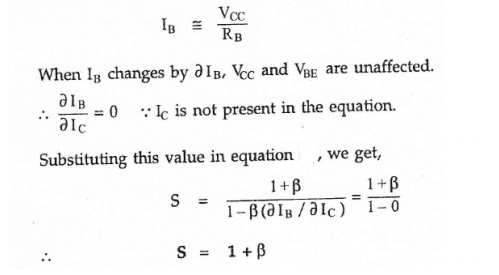Merits:

·        It is simple to shift the operating point anywhere in the active region by merely changing the base resistor (RB).

·        A very small number of components are required.

Demerits:

·        The collector current does not remain constant with variation in temperature or power supply voltage. Therefore the operating point is unstable.

·        Changes in Vbe will change IB and thus cause RE to change. This in turn will alter the gain of the stage.

·        When the transistor is replaced with another one, considerable change in the value of β can be expected. Due to this change the operating point will shift.

·        For small-signal transistors (e.g., not power transistors) with relatively high values of β (i.e., between 100 and 200), this configuration will be prone to thermal runaway. In particular, the stability factor, which is a measure of the change in collector current with changes in reverse saturation current, is approximately β+1. To ensure absolute stability of the amplifier, a stability factor of less than 25 is preferred, and so small-signal transistors have large stability factors.

Usage:

Due to the above inherent drawbacks, fixed bias is rarely used in linear circuits (i.e., those circuits which use the transistor as a current source). Instead, it is often used in circuits where transistor is used as a switch. However, one application of fixed bias is to achieve crude automatic gain control in the transistor by feeding the base resistor from a DC signal derived from the AC output of a later stage.

Problems

1. Design the fixed bias circuit from the load line given in the figure.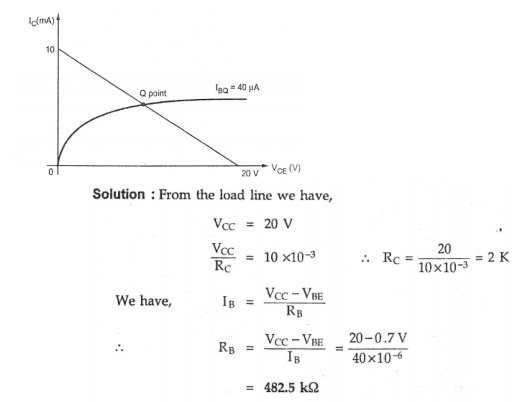2. For the circuit shown in figure. Calculate IB,IC,VCE,VB,VC and VBC. Assume VBE= 0.7V and β=50.3. Design a fixed biased circuit using a silicon transistor having β value of 100. Vcc is 10 V and dc bias conditions are to be VCE = 5 V and IC = 5 mA,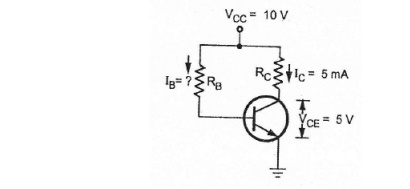Solution

Applying KVL to collector circuit,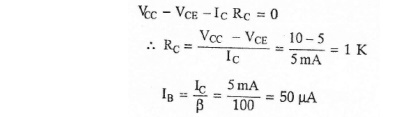Applying KVL to base circuit,4. Calculate the operating point (Q-point)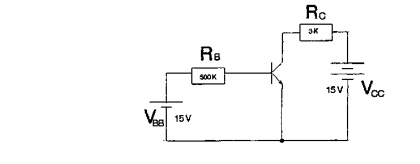Base biased CE connectionIC = βdc * IB = 100 * 29µA = 2.9 mA

VCE = VCC - (IC * RC) = 15V - (2.9 mA * 3KΩ) = 6.3V

By plotting IC (2.9 mA) and VCE (6.3V), we get the operation point ----> Q-point (quiescent point).Collector curve with load line and Q – point

5. Draw the load line and Q-point.base biased CE connection, β=50

Solution:IC = IB * β = 2.15 mA

VCE = VCC - (RC * IC)= 5.7V

`                                     VCE (cut) = VCC = 3.0VStudy Material, Lecturing Notes, Assignment, Reference, Wiki description explanation, brief detail
Electronic Circuits : Biasing of Discrete BJT and MOSFET : Fixed Bias (Base Resistor Bias) |